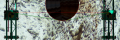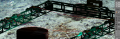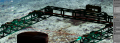# How can I detect a tangent common to two circles?

#### strantor

Joined Oct 3, 2010
5,324
This question is heavily math and heavily Python programming. If you have some input on the math but not the Python, that's still very welcome - I posted this in the math section because I don't want to limit it to a discussion of Python.

This is a real-world problem, not homework help. I'm using Blender to make a video game, and blender games are scripted in Python. In my video game I want to render a wire (just a line) wrapping around some sheave/pulleys and a large circle. The lines (including circles) must be drawn using X,Y coordinates. Sorry, no vectors or rays or curves or anything like that.

I can draw circles (24 sided polygons), arcs/hemicircles/quadrants (fractional 24-sided polygons), and straight lines with the X,Y method, but I cannot figure out how to detect the point on a circle where the wire should land, as if the wire were rested on the circumference of it.

Here's a visual:I want the red line to come up from left (just as it does), wrap around the left side wheel (just as it does), and then instead of continuing over to the right wheel (just as it does), it should go down around the circumference of the center circle and back up to the right wheel (just as the green dashed line does).

In order to do this, I need to know the line which is tangent to the left wheel and the center circle (and same for the right side). This will allow me to continue the arc around the left wheel >90 degrees (as shown, fixed 90deg) to the tangent point, draw the tangent, and begin another arc at the tangent point of the center circle, and so on, completing the wire loop.

I cannot use fixed coordinates, as the system is dynamic
Radius of left & right wheel is constant
Radius of the center circle is variable, and will get smaller during gameplay.
Y-value of the left & right wheels is variable.
Y-value of the center circle is variable.

If you have any input on the math, please chime in now.
If you have any Python experience, please check out the code below (the code used to generate the lines in the above image) and chime in if you have any input about that.

Thanks!

Code:
import bge
import math
cont = bge.logic.getCurrentController()
obj = cont.owner
sce = obj.scene
objects = sce.objects

RGB = [0.0,1.0,0.0]
GBR = [1.0,0.0,0.0]

#         Y is FWD/BACK (up & down the track)
#         X is left & right (port = neg, stbd = pos)
# circles start from STBD side and draw CCW; I use negative numbers & subtraction to make them draw CW

#3-Dimensional cartesian distance formula
def getdist(Xa,Ya,Za,Xb,Yb,Zb):
Xs = (Xa-Xb)**2
Ys = (Ya-Yb)**2
Zs = (Za-Zb)**2
return (math.sqrt(Xs+Ys+Zs))

#generates the coordinates for a catenary/parabolic droop given 2 end points
#Only in effect when the wire is slack; when tensioned, wire is straight
def create_catenary(amplitude,X1,Y1,Z1,X2,Y2,Z2,distance=0):
#DIVIDE THE SPAN OF THE LENGTH OF PORT SIDE INTO 100 SEGMENTS
#AND GENERATE A 100 SEGMENT LINE BETWEEN THE TWO POINTS
if distance == 0:
dist = getdist(X1,Y1,Z1,X2,Y2,Z2)
else:
dist = distance
y_change = (Y2-Y1)/100
x_change = (X2-X1)/100
for i in range(1,101):
deg1 = i * 1.78218 #180 degrees, divided by 101 segments
deg2 = (i + 1) * 1.78218
Y = Y1 + (y_change * i)
X = X1 + (x_change * i)
point_1 = [X,Y,Z]
Y = Y1 + (y_change * i) - y_change
X = X1 + (x_change * i) - x_change
point_2 = [X,Y,Z]
bge.render.drawLine(point_1, point_2, GBR)

flywheel_offset = .18

X1_port, Y1_port, Z1_port = objects["PORT AFT FLY"].worldPosition
X2_port, Y2_port, Z2_port = objects["PORT FWD FLY"].worldPosition
X1_fwd, Y1_fwd, Z1_fwd = objects["PORT FWD FLY"].worldPosition
X2_fwd, Y2_fwd, Z2_fwd = objects["STBD FWD FLY"].worldPosition
X1_stbd, Y1_stbd, Z1_stbd = objects["STBD FWD FLY"].worldPosition
X2_stbd, Y2_stbd, Z2_stbd = objects["STBD AFT FLY"].worldPosition
X1_aft, Y1_aft, Z1_aft = objects["STBD AFT FLY"].worldPosition
X2_aft, Y2_aft, Z2_aft = objects["PORT AFT FLY"].worldPosition

#center circle/hemicircle
start_angle = 2 * math.pi
sides = 24
angle_increment = 2 * math.pi / sides

center_x = objects["PYLON"].worldPosition.x
center_y = objects["PYLON"].worldPosition.y
center_z = Z1_fwd

end_angle = 1 * math.pi
while start_angle > end_angle:
bge.render.drawLine([circ_x1,circ_y1,center_z],[circ_x2,circ_y2,center_z],GBR)
start_angle -= angle_increment

start_angle = 1 * math.pi
sides = 24
angle_increment = 2 * math.pi / sides

center_x = X1_fwd
center_y = Y1_fwd
center_z = Z1_fwd

end_angle = .5 * math.pi
while start_angle > end_angle:
bge.render.drawLine([circ_x1,circ_y1,center_z],[circ_x2,circ_y2,center_z],GBR)
start_angle -= angle_increment

X1_port -=flywheel_offset
X2_port -=flywheel_offset
port_dist = getdist(X1_port,Y1_port,Z1_port,X2_port,Y2_port,Z2_port)
Y1_fwd +=flywheel_offset
Y2_fwd +=flywheel_offset
fwd_dist = getdist(X1_fwd,Y1_fwd,Z1_fwd,X2_fwd,Y2_fwd,Z2_fwd)
X1_stbd +=flywheel_offset
X2_stbd +=flywheel_offset
stbd_dist = getdist(X1_stbd,Y1_stbd,Z1_stbd,X2_stbd,Y2_stbd,Z2_stbd)
Y1_aft -=flywheel_offset
Y2_aft -=flywheel_offset
aft_dist = getdist(X1_aft,Y1_aft,Z1_aft,X2_aft,Y2_aft,Z2_aft)

#determine whether to slack wire or not
# (if distance between pulleys is less than wire loop length)
total_dist = port_dist +\
stbd_dist+\
fwd_dist+\
aft_dist+\
(flywheel_offset*4)
print(total_dist)
loop_length = 20.0790

if total_dist < loop_length:
amplitude = (total_dist - loop_length) * .5
else:
amplitude = 0
print(amplitude)

create_catenary(amplitude, X1_port, Y1_port, Z1_port, X2_port, Y2_port, Z2_port, port_dist)
create_catenary(amplitude, X1_fwd, Y1_fwd, Z1_fwd, X2_fwd, Y2_fwd, Z2_fwd, fwd_dist)
create_catenary(amplitude, X1_stbd, Y1_stbd, Z1_stbd, X2_stbd, Y2_stbd, Z2_stbd, stbd_dist)
create_catenary(amplitude, X1_aft, Y1_aft, Z1_aft, X2_aft, Y2_aft, Z2_aft, aft_dist)
The screenshot above will probably leave you wondering about all the "catenary" business in my code. For a visual explanation, see the pics below. The "wire" is a continuous loop which can be tightened/loosened by driving the wheels along the track in opposite directions.#### strantor

Joined Oct 3, 2010
5,324
Thank you wBahn. That looks very familiar. My pile of scratch papers have all those same lines drawn on them but with different trig functions. I think I'm just taking the scenic route to where you are, and get the same answers. But I am working a different way to get the X/Y coordinates out of it. That's the whole point; just looking for the most efficient way to get dots that I can plot. That's still got me chasing my tail. My trig is weak but I'll get it. I can get some of the trig functions to return coordinates but they're messed up, I guess because the trig functions assume a 0,0 starting point and a right triangle aligned to X & Y. Trying to correct them for orientation and position is making my brain hurt.

#### KL7AJ

Joined Nov 4, 2008
2,225
Thank you wBahn. That looks very familiar. My pile of scratch papers have all those same lines drawn on them but with different trig functions. I think I'm just taking the scenic route to where you are, and get the same answers. But I am working a different way to get the X/Y coordinates out of it. That's the whole point; just looking for the most efficient way to get dots that I can plot. That's still got me chasing my tail. My trig is weak but I'll get it. I can get some of the trig functions to return coordinates but they're messed up, I guess because the trig functions assume a 0,0 starting point and a right triangle aligned to X & Y. Trying to correct them for orientation and position is making my brain hurt.
I believe you could also use the parametric equations. I'll have to think on this. Great problem!

#### WBahn

Joined Mar 31, 2012
26,074
You need the points at which the red line L touches the circles. Let's call these <x3,y4> on the left and <x4,y4>on the right.

x3 = x1 + R1·sin('a')
y3 = y1 + R1·cos('a')

x4 = x2 - R2·sin('a')
y4 = y2 - R2·cos('a')

This assumes that x increases to the right and y increases upward. It also assumed that the tangent line goes from the top of the left circle to the bottom of the right circle (which is why it is addition in the first pair of equations and subtraction in the second). For your problem, there is also the assumption that Y2 < Y1 + (R1+R2) otherwise the line will not touch the second circuit at all, but rather just go straight across).

You can use symmetry to find the points for the right hand tangent line provided that symmetry applies. If not, it is easy enough to adjust the equations for the case of going from the bottom of the left circle to the top of the right circle.

•strantor

#### strantor

Joined Oct 3, 2010
5,324
You need the points at which the red line L touches the circles. Let's call these <x3,y4> on the left and <x4,y4>on the right.

x3 = x1 + R1·sin('a')
y3 = y1 + R1·cos('a')

x4 = x2 - R2·sin('a')
y4 = y2 - R2·cos('a')

This assumes that x increases to the right and y increases upward. It also assumed that the tangent line goes from the top of the left circle to the bottom of the right circle (which is why it is addition in the first pair of equations and subtraction in the second). For your problem, there is also the assumption that Y2 < Y1 + (R1+R2) otherwise the line will not touch the second circuit at all, but rather just go straight across).

You can use symmetry to find the points for the right hand tangent line provided that symmetry applies. If not, it is easy enough to adjust the equations for the case of going from the bottom of the left circle to the top of the right circle.
This works beautifully WBahn. You've saved me at least 100 mentamotional stress units. I love you.